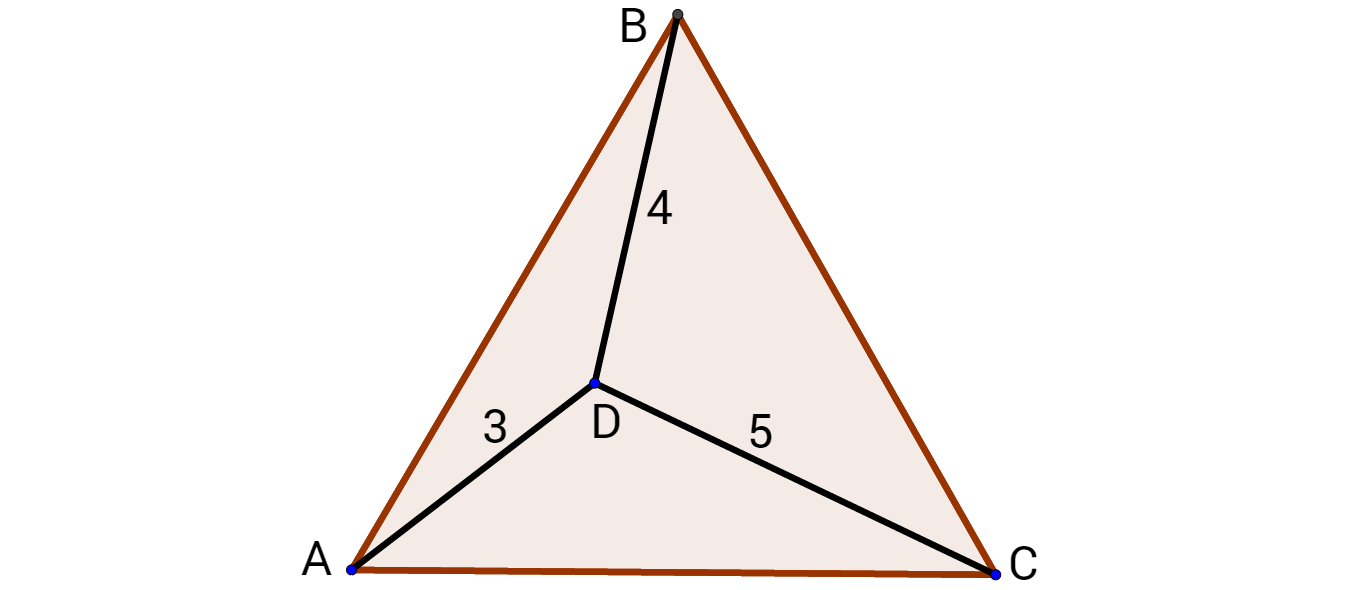They Look Like Pythagorean Triple

Geometry Level 4Triangle $ABC$ above is an equilateral triangle and $AD=3$, $BD=4$ and $CD=5$. Find $AB$.

×